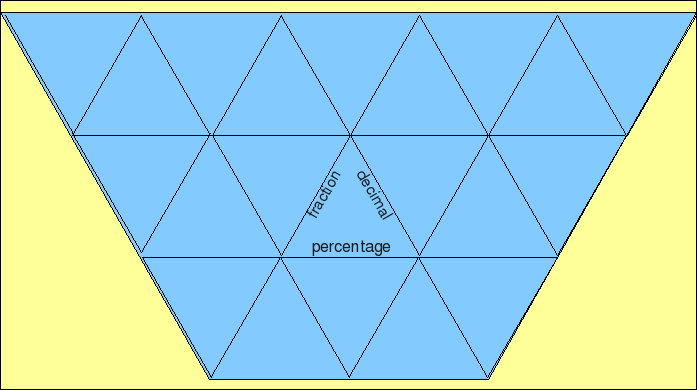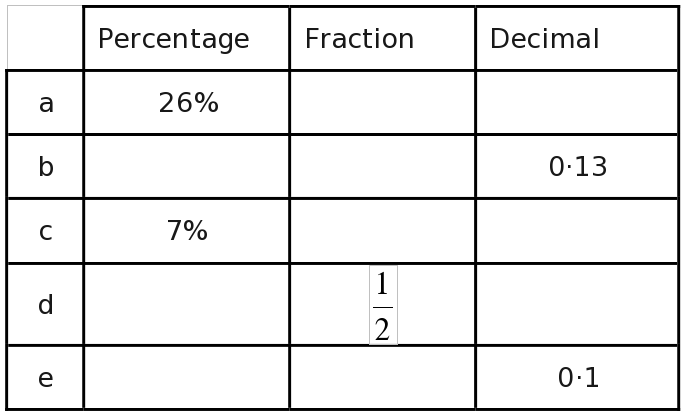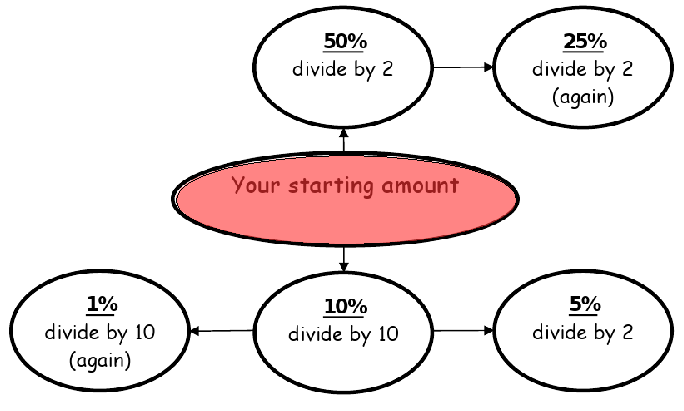## Last Week's Tests## Last Week's Tests

Did your test have any tables of numbers?

## Last Week's Tests

Did your test have any tables of numbers?
Do you think you found the right values to use?

## Last Week's Tests

Did your test have any tables of numbers?
Do you think you found the right values to use?
Were any strange words used in the questions?

## Last Week's Tests

Did your test have any tables of numbers?
Do you think you found the right values to use?
Were any strange words used in the questions?
Did you have to guess any words?

## Last Week's Tests

Did your test have any tables of numbers?
Do you think you found the right values to use?
Were any strange words used in the questions?
Did you have to guess any words?
What were the hardest questions?

## Last Week's Tests

Did your test have any tables of numbers?
Do you think you found the right values to use?
Were any strange words used in the questions?
Did you have to guess any words?
What were the hardest questions?
Which questions took too long?

Anything else?

## Joining the Cards## Session Topic:Finding Percentages Quickly## Percentages in the News?

Have we seen more "percentage" news than ever before?

## Percentages in the News?

Have we seen more "percentage" news than ever before?
What percent of MPs might be needed to call an early election?

## Percentages in the News?

Have we seen more "percentage" news than ever before?
What percent of MPs might be needed to call an early election?
What is going to happen to VAT?

## Percentages in the News?

Have we seen more "percentage" news than ever before?
What percent of MPs might be needed to call an early election?
What is going to happen to VAT?
Will you really notice the VAT change very much?

## Objectives

·Identify equivalent fractions, decimals & percentages

## Objectives

·Identify equivalent fractions, decimals & percentages

·Calculate percentages

## Objectives

·Identify equivalent fractions, decimals & percentages

·Calculate percentages

·Work out percentages quickly with divide by two and divide by ten

## Objectives

·Identify equivalent fractions, decimals & percentages

·Calculate percentages

·Work out percentages quickly with divide by two and divide by ten

·Describe different ways to calculate percentages

## One Way to Calculate Percentages

First, get a single percent...

## Helpful?

Decimal place value sheets are available...

## In the Worksheet...

A Table!## A Very Useful System!## Using the System...## Too many Questions?

Everyone should try the FIRST FOUR questions## Spot the Wrong Answer?

The First Question should NOT be 31

## Objectives

·Identify equivalent fractions, decimals & percentages

## Objectives

·Identify equivalent fractions, decimals & percentages

·Calculate percentages

## Objectives

·Identify equivalent fractions, decimals & percentages

·Calculate percentages

·Work out percentages quickly with divide by two and divide by ten

## Objectives

·Identify equivalent fractions, decimals & percentages

·Calculate percentages

·Work out percentages quickly with divide by two and divide by ten

·Describe different ways to calculate percentages

Thank you!

:
.

Thank you.

unhide